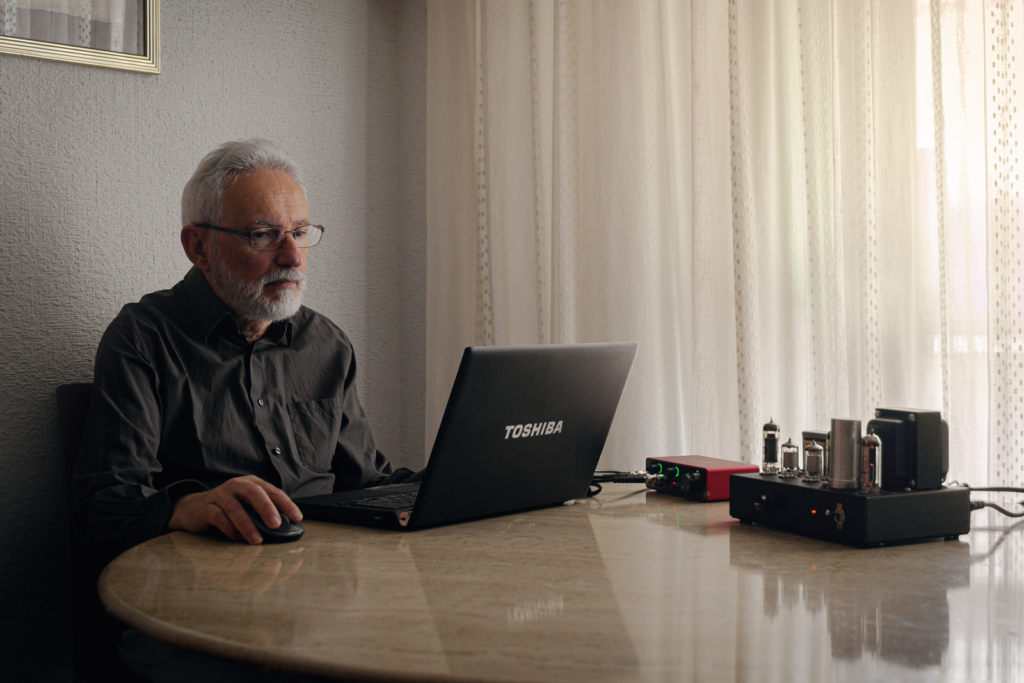# 1955 measurements

The measurements have been made after the circuit has been operated for one hour at room temperature approximately 25°C.

We have 290 V on the C9 filter capacitor instead of 310 V, maybe because our mains transformer has higher internal resistance than the one used in the Philips EZ81 graphs, which was our reference for the power supply calculations.

In my prototype I have tried using a higher voltage transformer, but the voltages of the circuit were too high, and I finally decided to use the 370DAX.

The output transformer also doesn’t have the same primary winding resistance as the one used in the original circuit. We have

Rp = (310 – 290) / 0.045 = 444 Ω

444 Ω in the original transformer versus 350 Ω measured in the 125BSE. It was necessary to change the value of the resistor R8 from 3.9 kΩ to 2.2 kΩ to equalize the plate and screen voltages of V2.

## Power consumption

Mains AC total power consumption was 245 mA over 230 V 50 Hz. That’s about 56 watts.

## DC Conditions

### Voltages

 Point of measurement Original 1955 Amp TR1 sec with no load 300 – 0 – 300 Vac 287 – 0 – 287 Vac TR1 sec loaded 270 – 0 – 270 Vac TR1 fil 6.3 Vac 6.3 Vac C9 310 Vdc 290 Vdc C6 290 Vdc 275 Vdc C3 210 Vdc 200 Vdc V2a 290 Vdc 275 Vdc V2s 290 vdc 275 Vdc V2k 28 Vdc 28 Vdc V1a 20 Vdc 20 Vdc V1s 28 Vdc 27 Vdc V1k 17.5 mVdc

### DC Currents (based on calculations from voltages)

 Point of measurement Original 1955 Amp R12 5.13 mA 6.82 mA V2a 45.08 mA 43.37 mA V2s 4.92 mA 6.63 mA V2k 50.00 mA 50.00 mA V1a 0.21 mA 0.19 mA V1k 0.21 mA Total per channel 50,21 mA 50,19 mA

## Performance

### Frequency Response & Phase Shift

The frequency-response characteristic for an output power of 0.3 W RMS is shown in the graphs below. It will be seen that the frequency response is level (to within 2 dB) from 20 Hz to 20 kHz. Measurements corresponding to the “improved circuit” graphs have been made without the feedback capacitor C5 and with the capacitor C8 capacity increased to 100 uF.

### Linearity

Relationship between input voltage and output power.### Sensitivity

The sensitivity of the amplifier measured at 1 kHz is 100 mV RMS for an output of 3.48 W RMS.

We can calculate the output power

P = U2 / RLOAD = 1322 / 5000 = 3.48 WRMS

For an input of 100 mV, the output is 132 V. Hence the output is 132 / 0.1 = 1320 times as great as the input (62 dB).

The gain of V1 is 3.33 / 0.1 = 33.3 times (30 dB)

the gain of V2 is 132 / 3.33 = 39.64 times (32 dB)

and the total voltage gain is 33.3 x 39.64 = 1320 times (30 + 32 = 62 dB)

When we increase the input voltage, we will have great distortion already in the first tube V1 as you can see in the following graphRicardo Pastor measuring the performance of the tube amplifier 1955